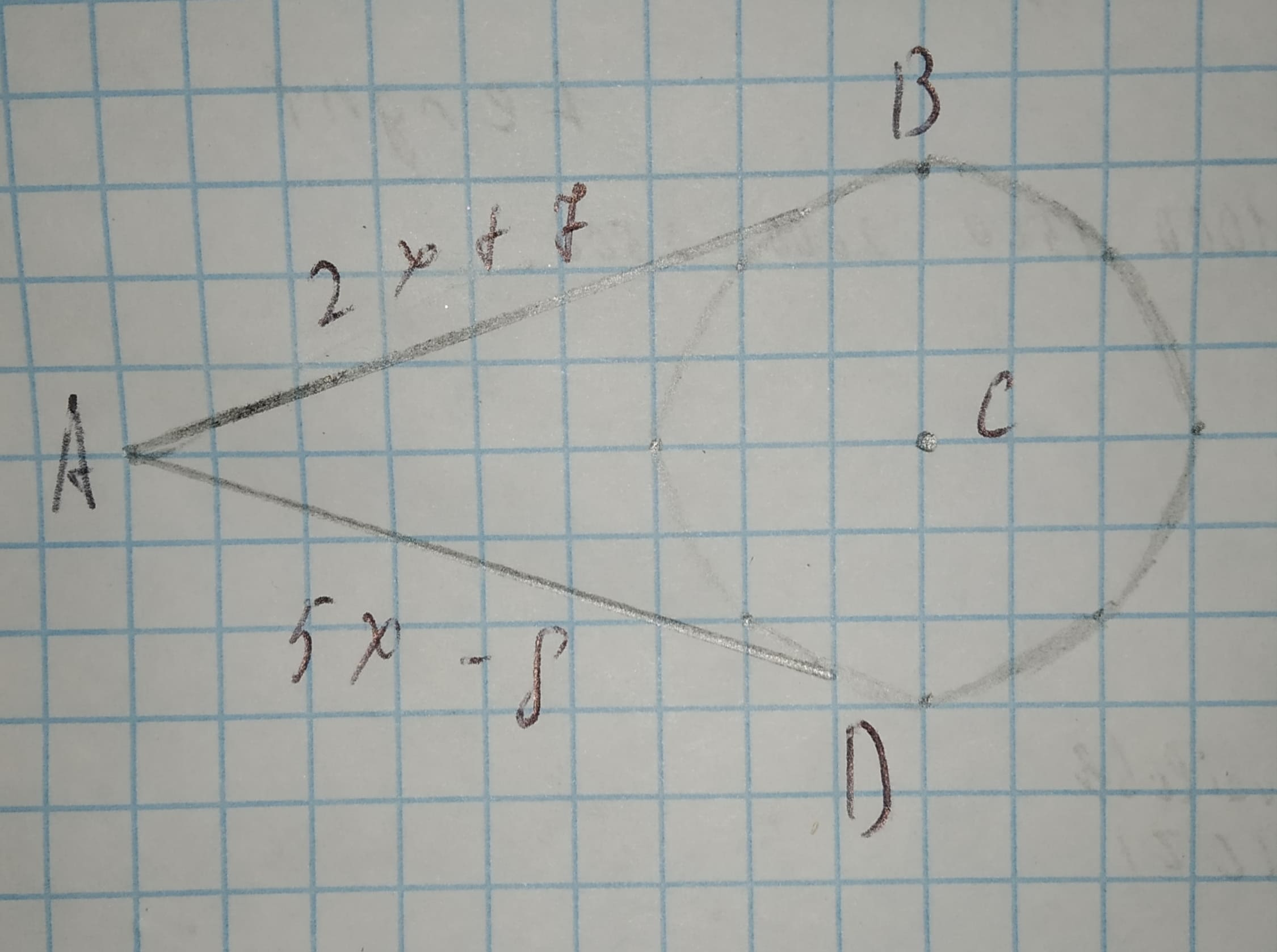# Points B and D are points of tangency. Find the value of x.12210204021.jpgEfan Halliday 2020-12-24 Answered

Points B and D are points of tangency. Find the value of x.You can still ask an expert for help

• Questions are typically answered in as fast as 30 minutes

Solve your problem for the price of one coffee

• Math expert for every subject
• Pay only if we can solve itunett
If from one external point, two tangents are drawn to a circle then they have equal tangent segments.
So, we can equate:
2x+7=5x-8
2x=5-15
-3x=-15
x=3
###### Not exactly what you’re looking for?Jeffrey Jordon